Next: Stationary states Up: Quantum Mechanics Previous: Anomalous Zeeman effect

### Schrodinger's equation

We have already introduced ourselves to the wavefunction. Recall that the wave function is not only a function of the spatial coordinates of the particles but also a function of time. In order to follow the dynamics of the system we need to follow the temporal evolution of the wave function. This may be done by solving an equation formulated by Schrodinger, better known as the time dependent Schrodinger equation: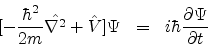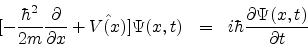V(x) is the time independent potential energy operator for the system in question which in turn is not subjected to any time dependent external force.

For such a system we may write the wavefunctionas a product of a spatial part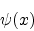and a temporal part.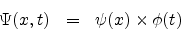This separation of variable technique separates the time dependent Schrodinger equation into a temporal equation whose solution gives us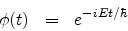and a space dependent equation which is nothing but the time independent Schrodinger equation.

E is postulated to be the energy of the system which is a real constant.

The solution of the above equation would yield. Hencecan then be obtained as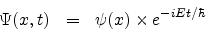.

SubsectionsNext: Stationary states Up: Quantum Mechanics Previous: Anomalous Zeeman effect
Abhijit Poddar
2007-09-27